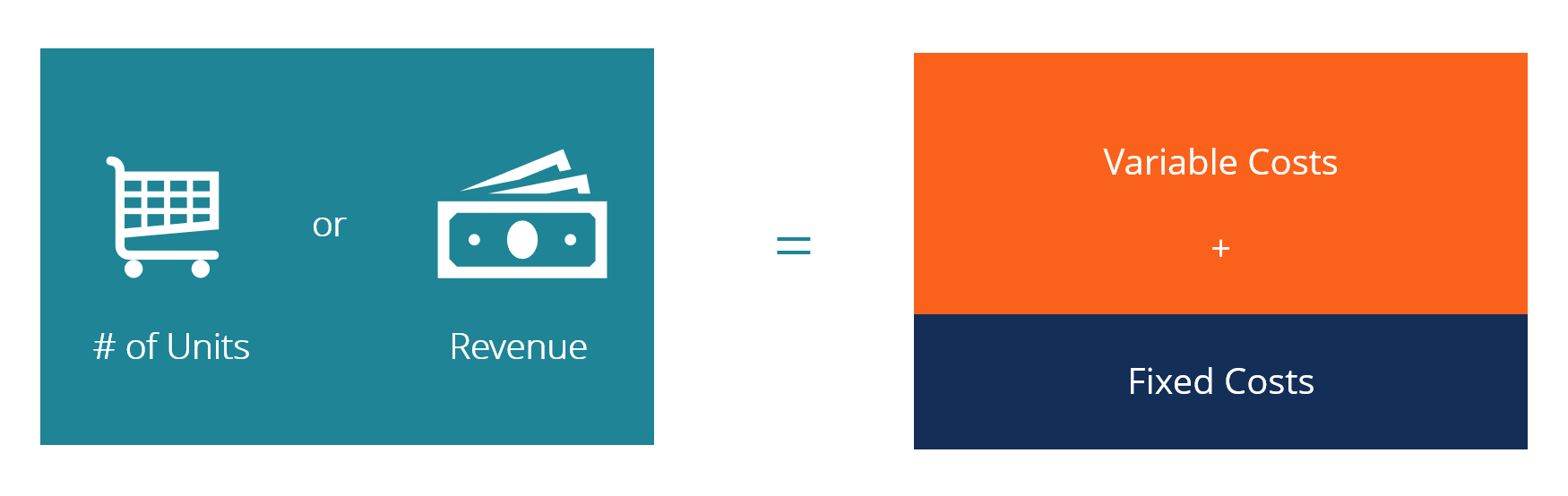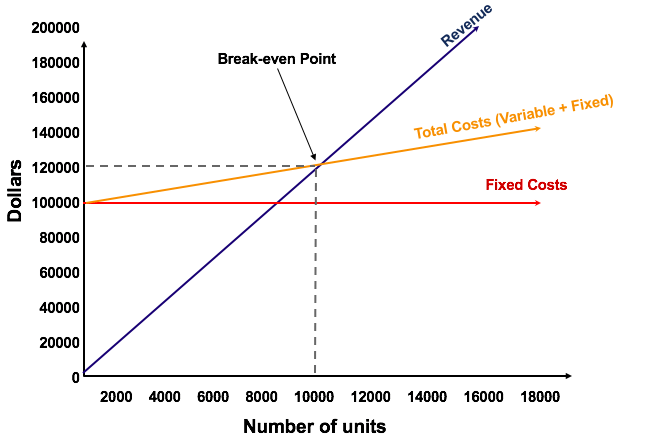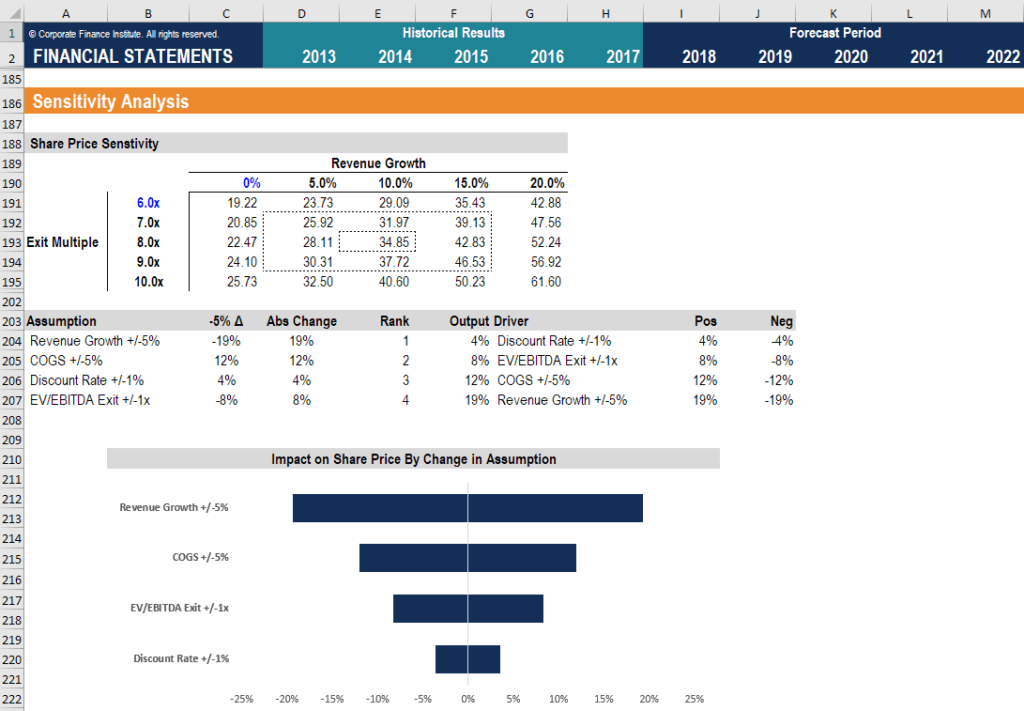# Break Even Analysis

The point in which total cost and total revenue are equal

## What is Break Even Analysis?

Break Even Analysis in economics, business, and cost accounting refers to the point in which total cost and total revenue are equal. A break even point analysis is used to determine the number of units or dollars of revenue needed to cover total costs (fixed and variable costs).Image: CFI’s Budgeting & Forecasting Course.

### Formula for Break Even Analysis

The formula for break even analysis is as follows:

Break even quantity = Fixed costs / (Sales price per unit – Variable cost per unit)

Where:

• Fixed costs are costs that do not change with varying output (i.e. salary, rent, building machinery).
• Sales price per unit is the selling price (unit selling price) per unit.
• Variable cost per unit is the variable costs incurred to create a unit.

It is also helpful to note that sales price per unit minus variable cost per unit is the contribution margin per unit. For example, if a book’s selling price is \$100 and its variable costs are \$5 to make the book, \$95 is the contribution margin per unit and contributes to offsetting the fixed costs.

### Example of Break Even Analysis

Colin is the managerial accountant in charge of Company A, which sells water bottles. He previously determined that the fixed costs of Company A consist of property taxes, a lease, and executive salaries, which add up to \$100,000. The variable costs associated with producing one water bottle is \$2 per unit. The water bottle is sold at a premium price of \$12. To determine the break even point of Company A’s premium water bottle:

Break even quantity = \$100,000 / (\$12 – \$2) = 10,000

Therefore, given the fixed costs, variable costs, and selling price of the water bottles, Company A would need to sell 10,000 units of water bottles to break even.

### Graphically Representing the Break Even Point

The graphical representation of unit sales and dollar sales needed to break even is referred to as the break even chart or Cost Volume Profit (CVP) graph. Below is the CVP graph of the example above:#### Explanation:

1. The number of units is on the X-axis (horizontal) and the dollar amount is on the Y-axis (vertical).
2. The red line represents the total fixed costs of \$100,000.
3. The blue line represents revenue per unit sold. For example, selling 10,000 units would generate 10,000 x \$12 = \$120,000 in revenue.
4. The yellow line represents total costs (fixed and variable costs). For example, if the company sells 0 units, the company would incur \$0 in variable costs but \$100,000 in fixed costs for total costs of \$100,000. If the company sells 10,000 units, the company would incur 10,000 x \$2 = \$20,000 in variable costs and \$100,000 in fixed costs for total costs of \$120,000.
5. The break even point is at 10,000 units. At this point, revenue would be 10,000 x \$12 = \$120,000 and costs would be 10,000 x 2 = \$20,000 in variable costs and \$100,000 in fixed costs.
6. When the number of units exceeds 10,000, the company would be making a profit on the units sold. Note that the blue revenue line is greater than the yellow total costs line after 10,000 units are produced. Likewise, if the number of units is below 10,000, the company would be making a loss. From 0-9,999 units, the total costs line is above the revenue line.### Interpretation of Break Even Analysis

As illustrated in the graph above, the point at which total fixed and variable costs equal to total revenues is known as the break even point. At the break even point, a business does not make a profit or loss. Therefore, the break-even point is often referred to as the ‘no-profit’ or ‘no-loss point.’

The break even analysis is important to business owners and managers in determining how many units (or revenues) are needed to cover fixed and variable expenses of the business.

Therefore, the concept of break even point is as follows:

• Profit when Revenue > Total Variable cost + Total Fixed cost
• Break-even point when Revenue = Total Variable cost + Total Fixed cost
• Loss when Revenue < Total Variable cost + Total Fixed cost

### Sensitivity Analysis

Break even analysis is often a component of sensitivity analysis and scenario analysis performed in financial modeling. Using Goal Seek in Excel, an analyst can backsolve how many units need to be sold, at what price, and at what cost to break even.Image: CFI’s Financial Modeling Course.